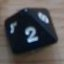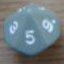Dark Coast dice pool

## Dark Coast dice pool

 Mon Oct 24 16:05:49 2011   by   Torben I'm working on a homebrew RPG called "Dark Coast".  You can find it at `http://www.diku.dk/~torbenm/DarkCoast/`.Dark Coast uses a rather unusual dice-pool system.  As usual, ability (talent level) is measure as a number of dice, but unlike other systems, so is difficulty (challenge level).  The system is fairly simple: At talent level T and challenge level C, you roll T+1+C d6s, drop the T lowest and take the now lowest as the result.  Equivalently, you can drop the C highest and take the now highest as the result -- you get the same number either way.  The resulting number (between 1 and 6) is interpreted as a degree of success, with 1-3 being various degrees of failure and 4-6 being degrees of success.With fixed C, the success chance increases with higher T and is exactly 50% if T=C.  But apart from the symmetric case, analysing probabilities by hand is not easy: Though you can find a closed formula for the probability of Nth-lowest of M dice being at least K, this is rather complex, so it is easier to use Troll.In Troll, you can simply write`min largest (C+1) (T+1+C)d6`where T is the talent level and C is the challenge level. There is no operator in Troll for dropping the T lowest dice, so I instead keep the C+1 largest, which is the same when the total is T+1+C.  Equivalently, you can write`max least (T+1) (T+1+C)d6`which drops the C largest dice and takes the largest of the remaining.If T>C, the first option is faster and if T